# Math Rotations | MathHelp.com

##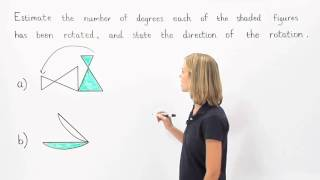By MathHelp.com

This lesson covers inverse variation. Students learn that inverse variation is a relationship between two variables in which one variable increases while the other variable decreases. And if the variables are x and y, the following formula can be used to represent inverse variation: xy = k, where k is the constant of variation. Students also learn that the following formula can be used to represent inverse variation between two ordered pairs: x1y1 = x2y2.# Inverse Variation | MathHelp.com

##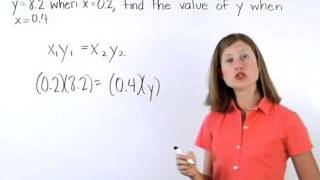By MathHelp.com

This lesson covers the surface area of a pyramid. Students learn the definition of a prism, as well as the following vocabulary words related to prisms: base, base edge, lateral edge, lateral face, and altitude (height). Students also learn the formulas for the lateral area, total area, and volume of a right prism, and are asked to solve problems using these formulas. Note that the prisms in this lesson are primarily rectangular prisms -- prisms whose bases are rectangles.# Inverse Variation | MathHelp.com

##By MathHelp.com# SAT Math Part 16 - Direct Variation Problems

##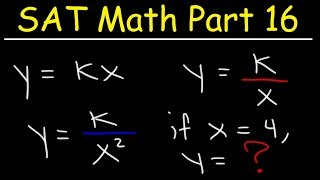By The Organic Chemistry Tutor# Inversion with Negative Adverbs - English Grammar with Jennifer

##By JenniferESL# Algebra Basics: Solving Basic Equations Part 2 - Math Antics

##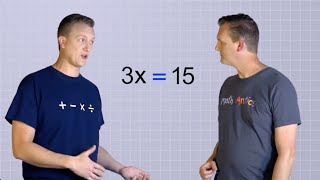By mathantics# Highlights from Jennifer's Patreon Member-only Playlist - JOIN today!

##By JenniferESL# Algebra Basics: Solving 2-Step Equations - Math Antics

##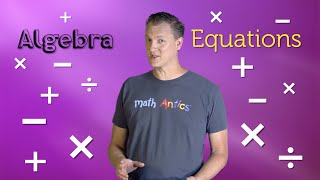By mathantics# Math Antics - Integer Multiplication & Division

##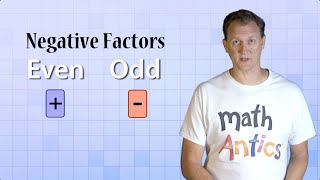By mathantics